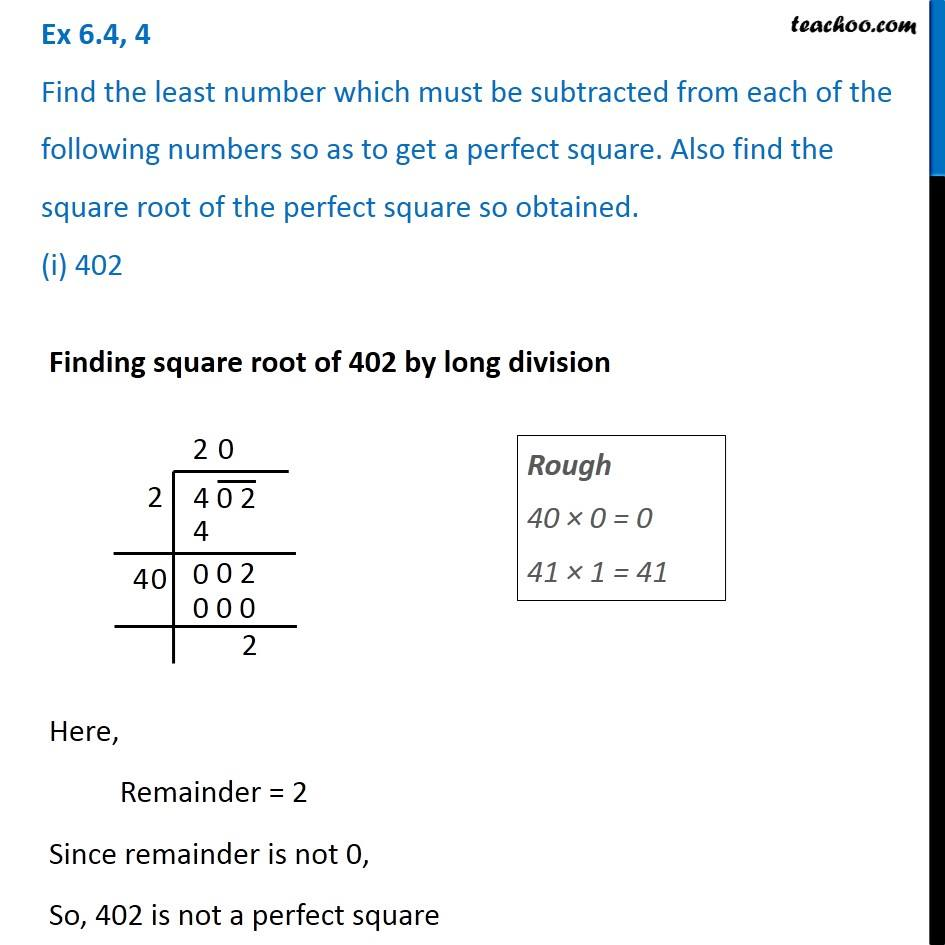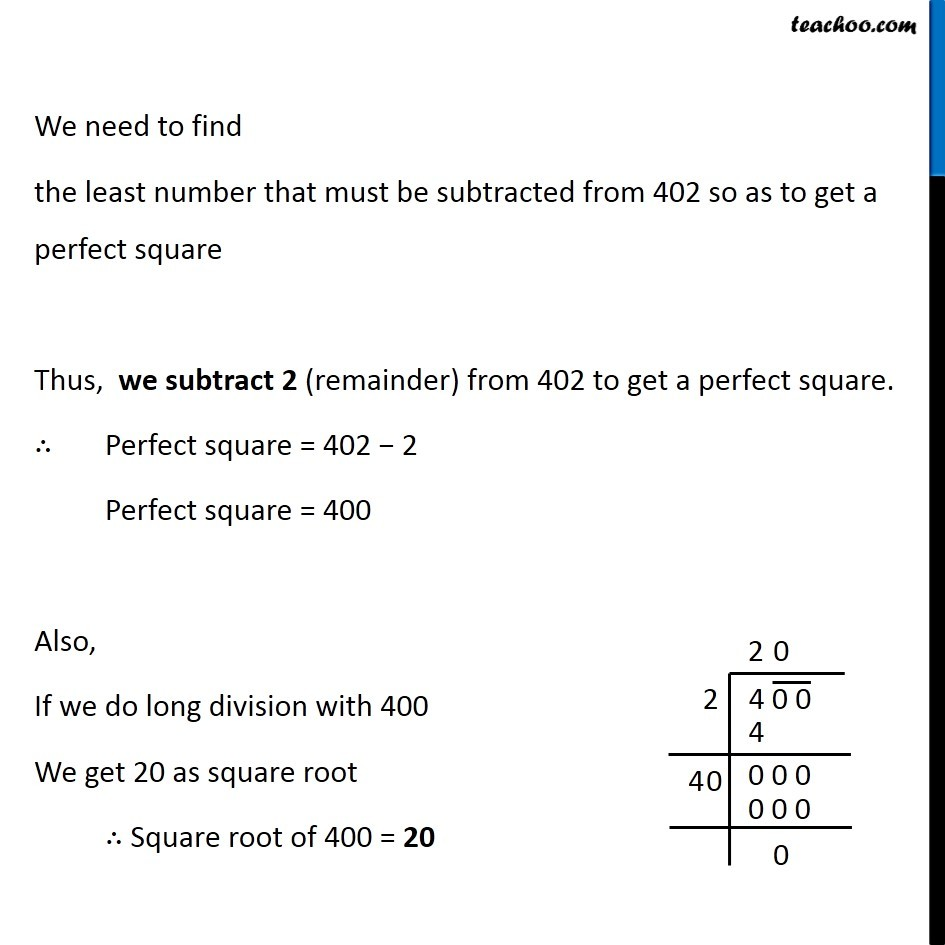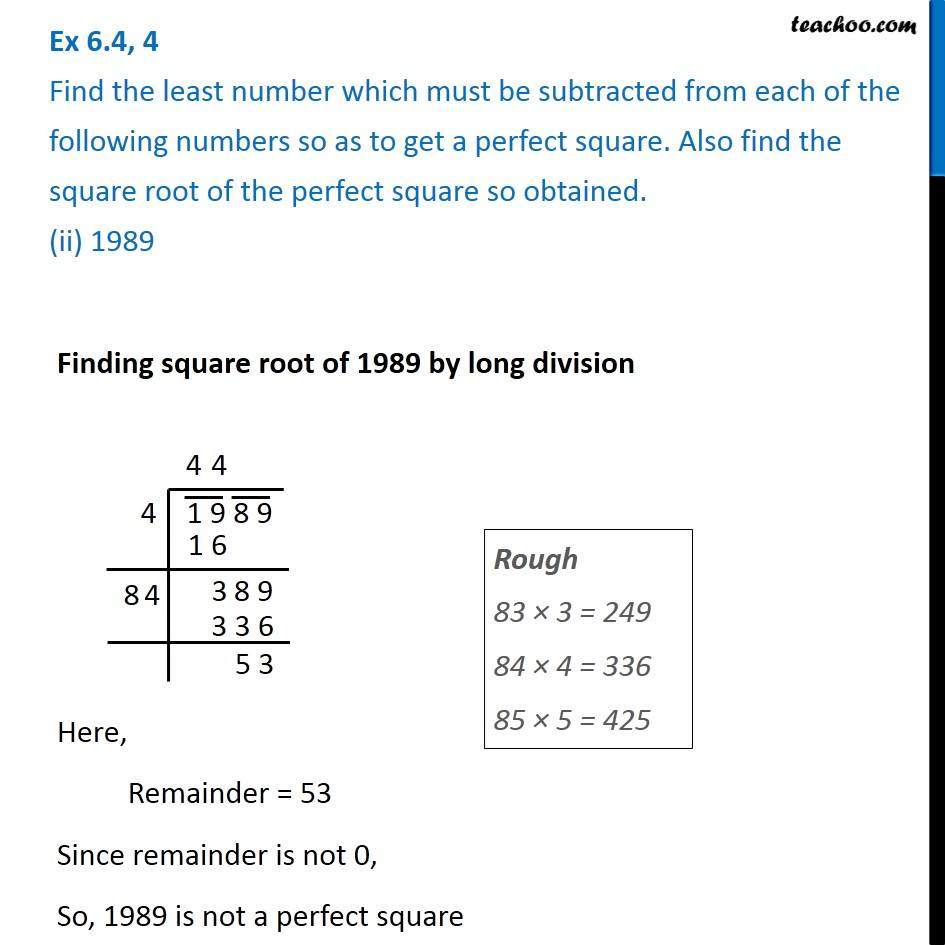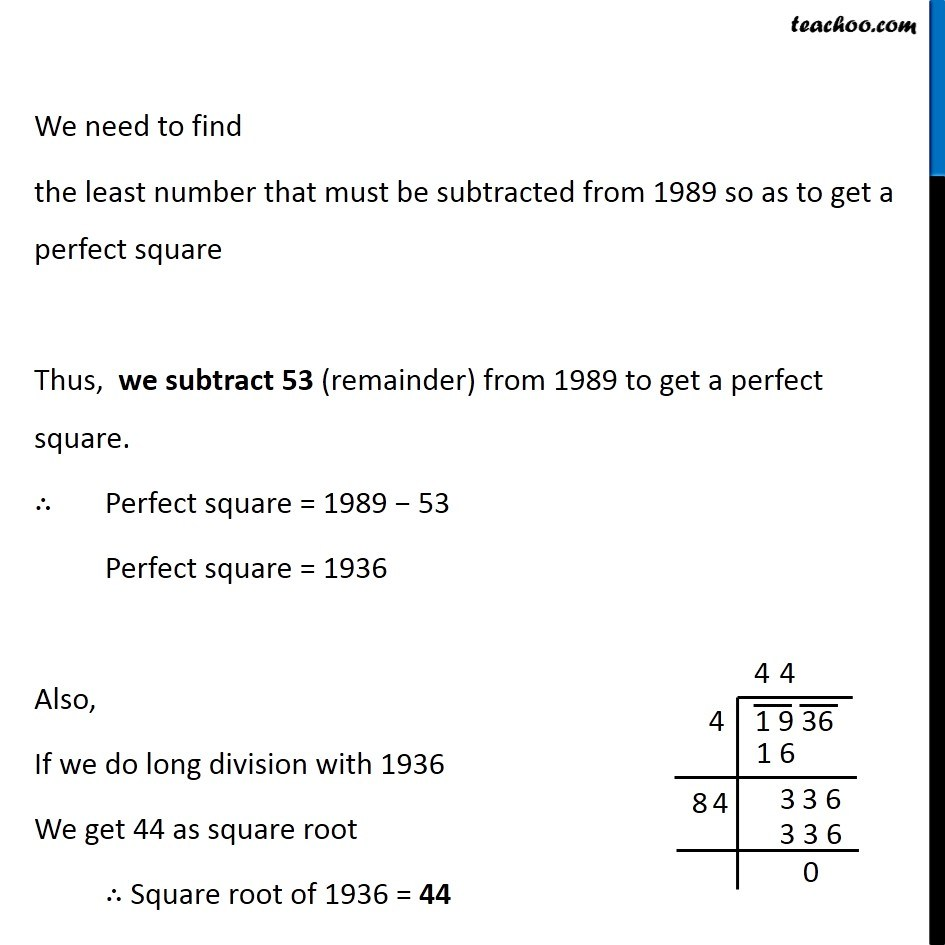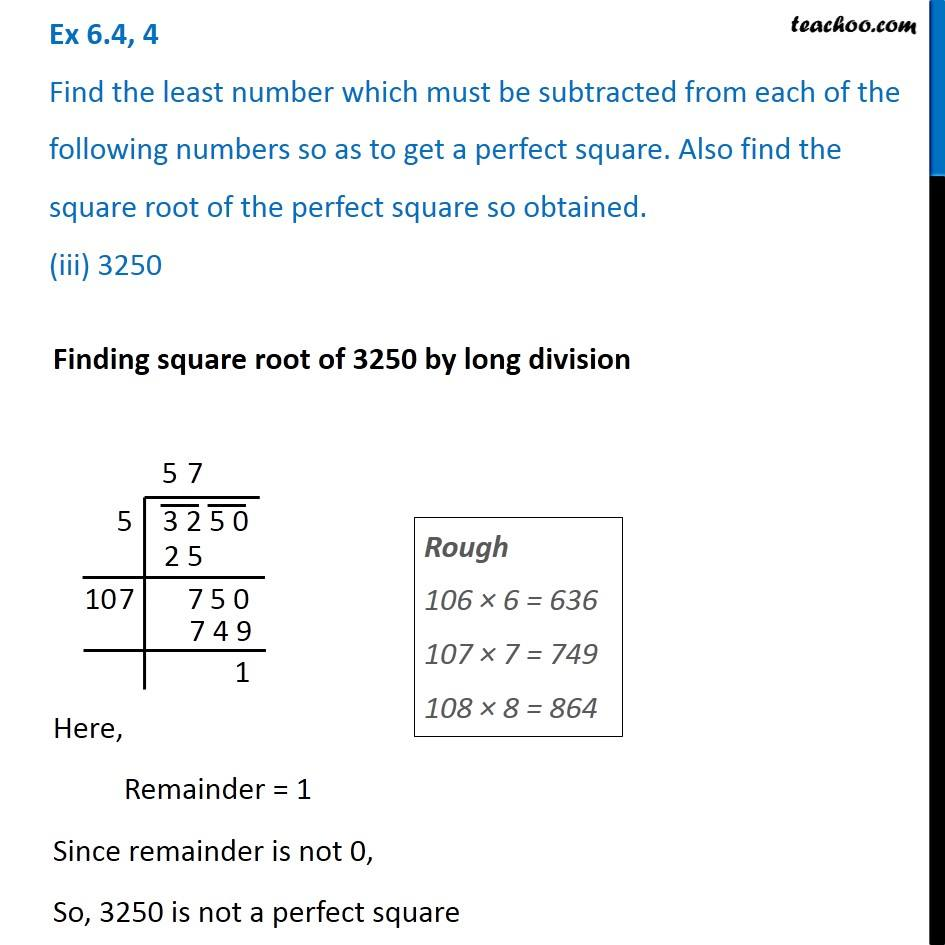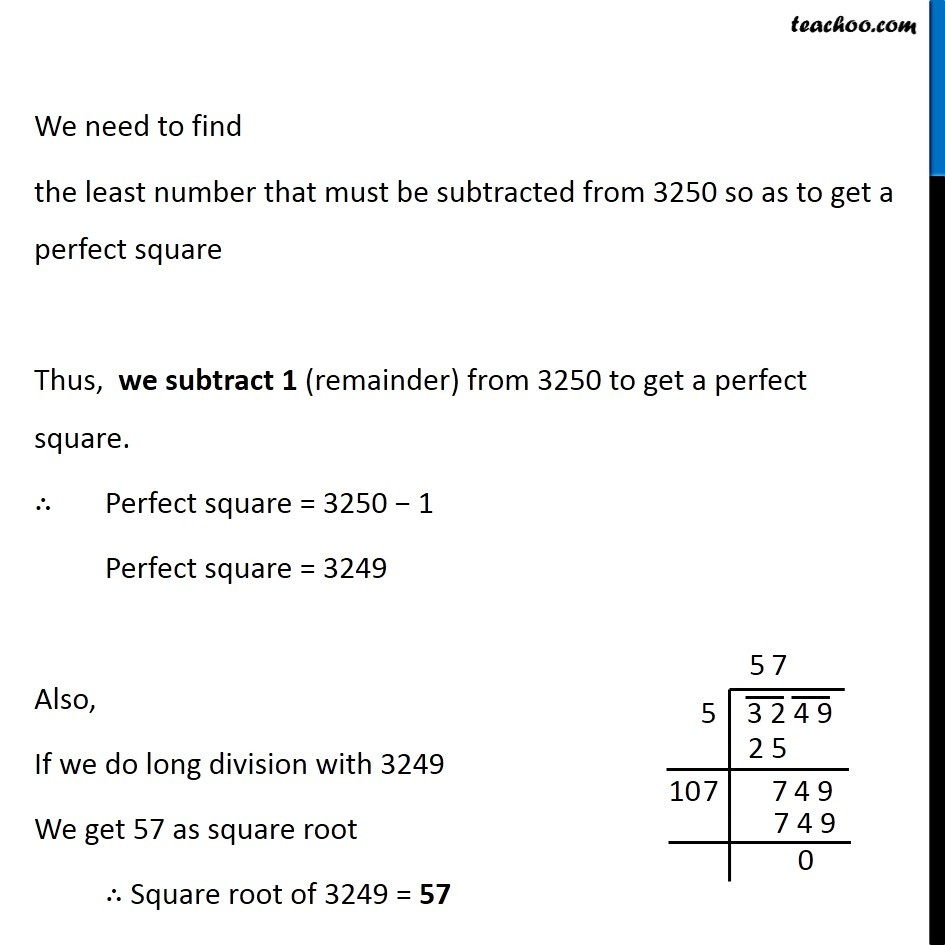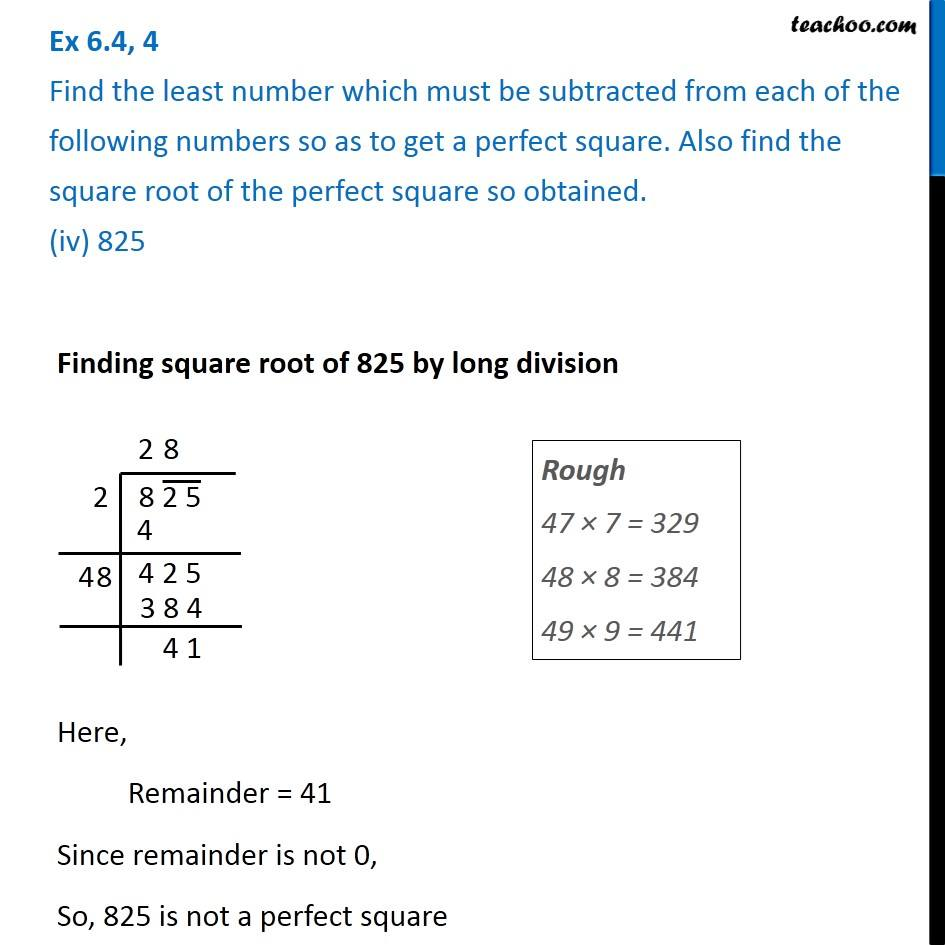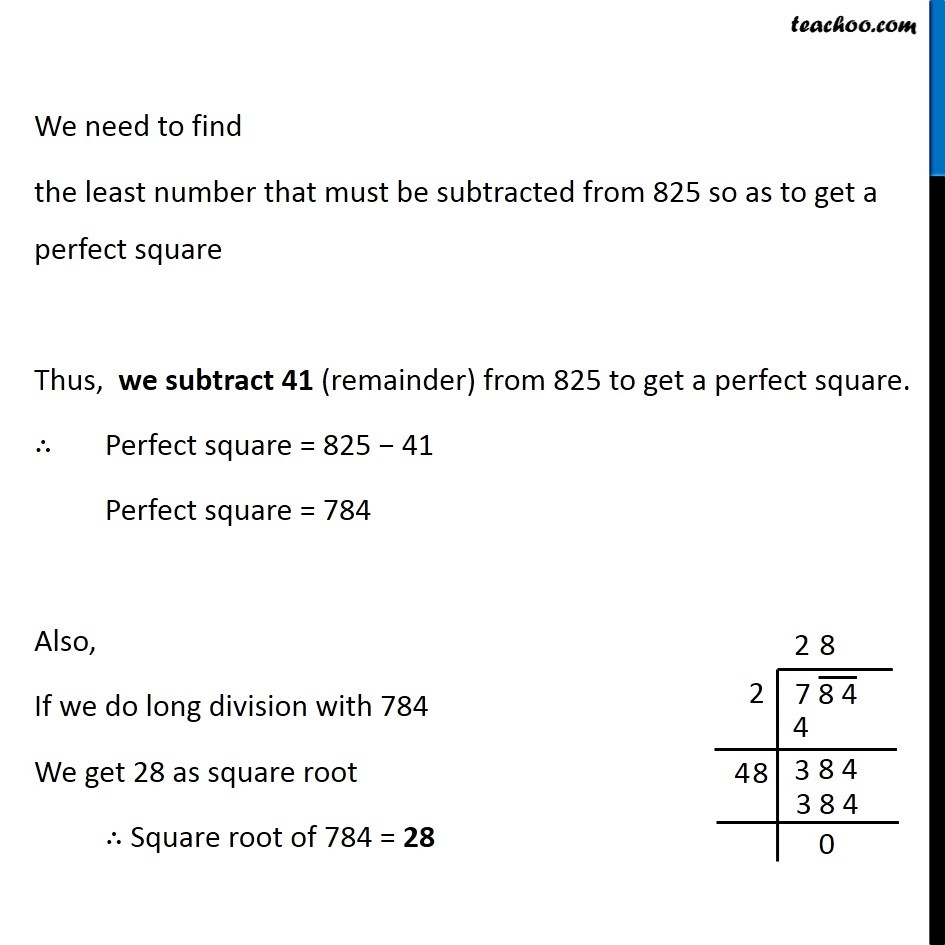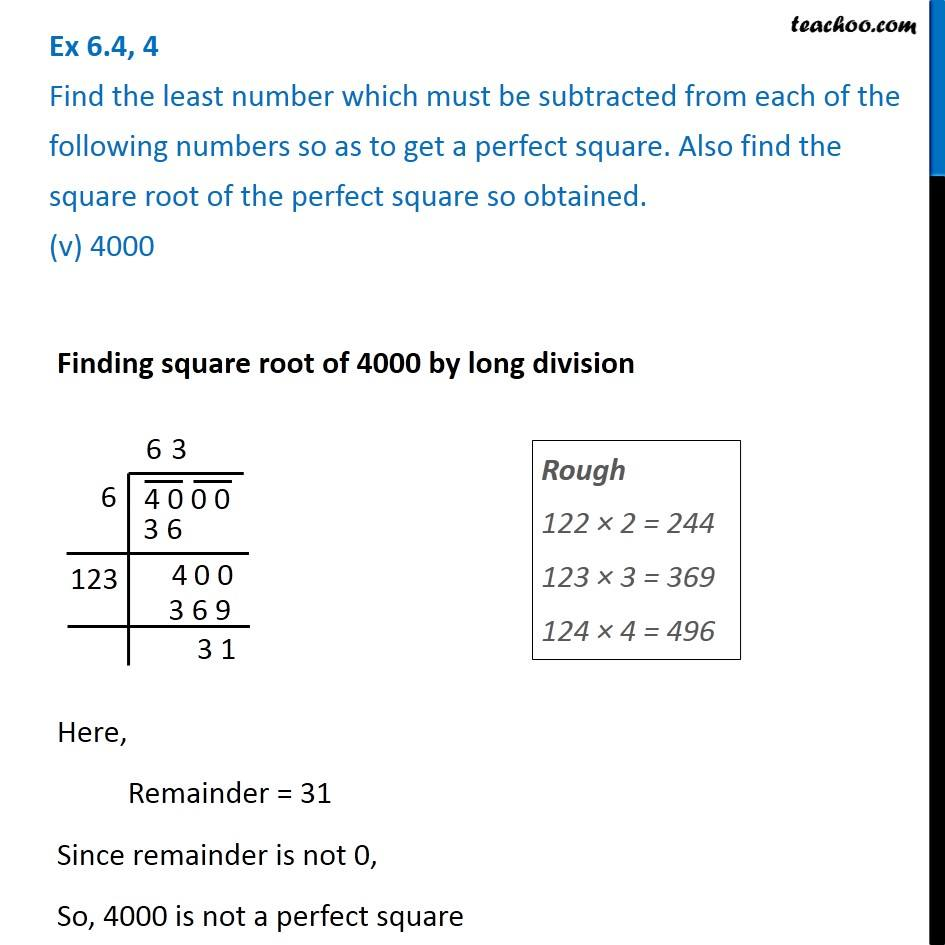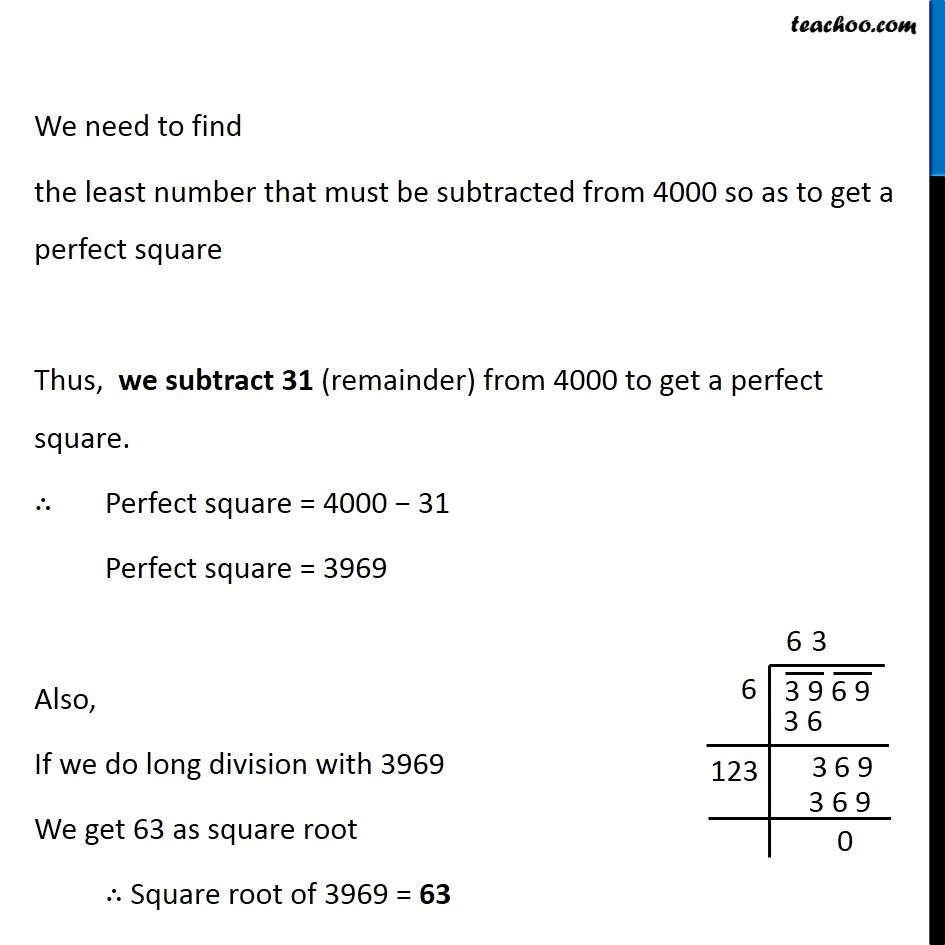Subscribe to our Youtube Channel - https://you.tube/teachoo

1. Chapter 6 Class 8 Squares and Square Roots
2. Serial order wise
3. Ex 6.4

Transcript

Ex 6.4, 4 Find the least number which must be subtracted from each of the following numbers so as to get a perfect square. Also find the square root of the perfect square so obtained. (i) 402 Finding square root of 402 by long division Here, Remainder = 2 Since remainder is not 0, So, 402 is not a perfect square Rough 40 × 0 = 0 41 × 1 = 41 We need to find the least number that must be subtracted from 402 so as to get a perfect square Thus, we subtract 2 (remainder) from 402 to get a perfect square. ∴ Perfect square = 402 − 2 Perfect square = 400 Also, If we do long division with 400 We get 20 as square root ∴ Square root of 400 = 20 Ex 6.4, 4 Find the least number which must be subtracted from each of the following numbers so as to get a perfect square. Also find the square root of the perfect square so obtained. (ii) 1989 Finding square root of 1989 by long division Here, Remainder = 53 Since remainder is not 0, So, 1989 is not a perfect square We need to find the least number that must be subtracted from 1989 so as to get a perfect square Thus, we subtract 53 (remainder) from 1989 to get a perfect square. ∴ Perfect square = 1989 − 53 Perfect square = 1936 Also, If we do long division with 1936 We get 44 as square root ∴ Square root of 1936 = 44 Ex 6.4, 4 Find the least number which must be subtracted from each of the following numbers so as to get a perfect square. Also find the square root of the perfect square so obtained. (iii) 3250 Finding square root of 3250 by long division Here, Remainder = 1 Since remainder is not 0, So, 3250 is not a perfect square Rough 106 × 6 = 636 107 × 7 = 749 108 × 8 = 864 We need to find the least number that must be subtracted from 3250 so as to get a perfect square Thus, we subtract 1 (remainder) from 3250 to get a perfect square. ∴ Perfect square = 3250 − 1 Perfect square = 3249 Also, If we do long division with 3249 We get 57 as square root ∴ Square root of 3249 = 57 Ex 6.4, 4 Find the least number which must be subtracted from each of the following numbers so as to get a perfect square. Also find the square root of the perfect square so obtained. (iv) 825 Finding square root of 825 by long division Here, Remainder = 41 Since remainder is not 0, So, 825 is not a perfect square We need to find the least number that must be subtracted from 825 so as to get a perfect square Thus, we subtract 41 (remainder) from 825 to get a perfect square. ∴ Perfect square = 825 − 41 Perfect square = 784 Also, If we do long division with 784 We get 28 as square root ∴ Square root of 784 = 28 Ex 6.4, 4 Find the least number which must be subtracted from each of the following numbers so as to get a perfect square. Also find the square root of the perfect square so obtained. (v) 4000 Finding square root of 4000 by long division Here, Remainder = 31 Since remainder is not 0, So, 4000 is not a perfect square Rough 122 × 2 = 244 123 × 3 = 369 124 × 4 = 496 We need to find the least number that must be subtracted from 4000 so as to get a perfect square Thus, we subtract 31 (remainder) from 4000 to get a perfect square. ∴ Perfect square = 4000 − 31 Perfect square = 3969 Also, If we do long division with 3969 We get 63 as square root ∴ Square root of 3969 = 63

Ex 6.4